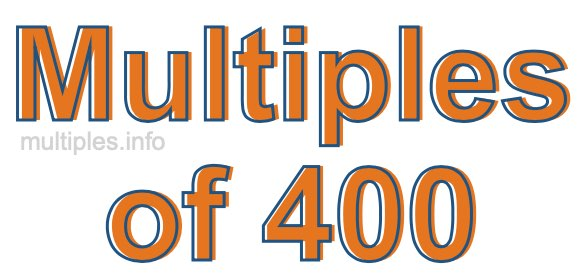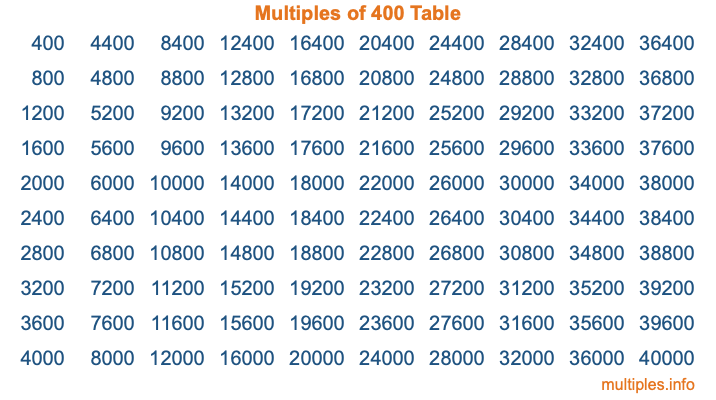Multiples of 400Welcome to the Multiples of 400 page. Here we will first teach you everything you will ever need to know about the multiples of 400, and then give you a study guide summary of everything we taught you to make sure you remember it all. Use this page to look up facts and learn information about the multiples of 400. This page will make you a multiples of four hundred expert!

Definition of Multiples of 400
Multiples of 400 are all the numbers that when divided by 400 equal an integer. Each of the multiples of 400 are called a multiple. A multiple of 400 is created by multiplying 400 by an integer.

Therefore, to create a list of multiples of 400, you start with 1 multiplied by 400, then 2 multiplied by 400, then 3 multiplied by 400, and so on for as long as you want. Thus, the list of the first five multiples of 400 is 400, 800, 1200, 1600, and 2000. To see a larger list of multiples of 400, see the printable image of Multiples of 400 further down on this page. We also have a category where you can choose any nth multiple of 400.

Multiples of 400 Checker
The Multiples of 400 Checker below checks to see if any number of your choice is a multiple of 400. In other words, it checks to see if there is any number (integer) that when multiplied by 400 will equal your number. To do that, we divide your number by 400. If the the quotient is an integer, then your number is a multiple of 400.

Is  a multiple of 400?

Least Common Multiple of 400 and ...
A Least Common Multiple (LCM) is the lowest multiple that two or more numbers have in common. This is also called the smallest common multiple or lowest common multiple and is useful to know when you are adding our subtracting fractions. Enter one or more numbers below (400 is already entered) to find the LCM.

Check out our LCM Calculator if you need more details about the Least Common Multiple or if you need the LCM for different numbers for adding and subtraction fractions.

nth Multiple of 400
As we stated above, 400 is the first multiple of 400, 800 is the second multiple of 400, 1200 is the third multiple of 400, and so on. Enter a number below to find the nth multiple of 400.

th multiple of 400

Multiples of 400 vs Factors of 400
400 is a multiple of 400 and a factor of 400, but that is where the similarities end. All postive multiples of 400 are 400 or greater than 400. All positive factors of 400 are 400 or less than 400.

Below is the beginning list of multiples of 400 and the factors of 400 so you can compare:

Multiples of 400: 400, 800, 1200, 1600, 2000, etc.

Factors of 400: 1, 2, 4, 5, 8, 10, 16, 20, 25, 40, 50, 80, 100, 200, 400

As you can see, the multiples of 400 are all the numbers that you can divide by 400 to get a whole number. The factors of 400, on the other hand, are all the whole numbers that you can multiply by another whole number to get 400.

It's also interesting to note that if a number (x) is a factor of 400, then 400 will also be a multiple of that number (x).

Multiples of 400 vs Divisors of 400
The divisors of 400 are all the integers that 400 can be divided by evenly. Below is a list of the divisors of 400.

Divisors of 400: 1, 2, 4, 5, 8, 10, 16, 20, 25, 40, 50, 80, 100, 200, 400

The interesting thing to note here is that if you take any multiple of 400 and divide it by a divisor of 400, you will see that the quotient is an integer.

Multiples of 400 Table
Below is an image of the first 100 multiples of 400 in a table. The table is in chronological order, column by column. The first column has the first ten multiples of 400, the second column has the next ten multiples of 400, and so on.The Multiples of 400 Table is also referred to as the 400 Times Table or Times Table of 400. You are welcome to print out our table for your studies.

Negative Multiples of 400
Although not often discussed or needed in math, it is worth mentioning that you can make a list of negative multiples of 400 by multiplying 400 by -1, then by -2, then by -3, and so on, to get the following list of negative multiples of 400:

-400, -800, -1200, -1600, -2000, etc.

Multiples of 400 Summary
Below is a summary of important Multiples of 400 facts that we have discussed on this page. To retain the knowledge on this page, we recommend that you read through the summary and explain to yourself or a study partner why they hold true.

There are an infinite number of multiples of 400.

A multiple of 400 divided by 400 will equal a whole number.

400 divided by a factor of 400 equals a divisor of 400.

The nth multiple of 400 is n times 400.

The largest factor of 400 is equal to the first positive multiple of 400.

400 is a multiple of every factor of 400.

400 is a multiple of 400.

A multiple of 400 divided by a divisor of 400 equals an integer.

400 divided by a divisor of 400 equals a factor of 400.

Any integer times 400 will equal a multiple of 400.

Multiples of a Number
Here you can get the multiples of another number, all with the same attention to detail as we did for multiples of 400 on this page.

Multiples of
Multiples of 401
Did you find our page about multiples of four hundred educational? Do you want more knowledge? Check out the multiples of the next number on our list!